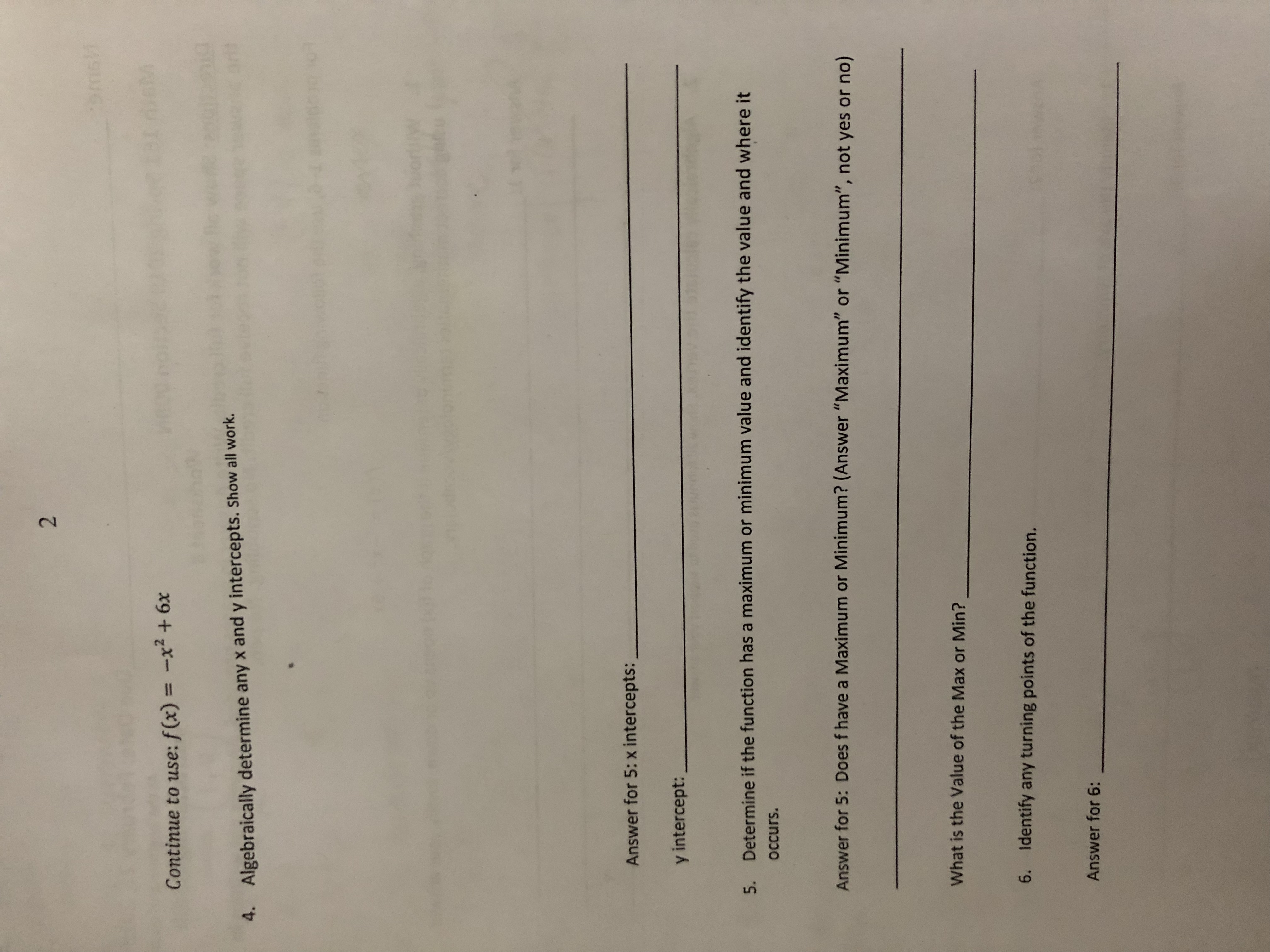# Continue to use: fCx)2+ 6x4.Algebraically determine any x and y intercepts. Show all work.Answer for 5: x intercepts:y intercept:Determine if the function has a maximum or minimum value and identify the value and where itoccurs.5.Answer for 5: Does f have a Maximum or Minimum? (Answer "Maximum" or "Minimum", not yes or no)What is the Value of the Max or Min?6.Identify any turning points of the function.Answer for 6

Question
26 views

Can you help me solve question 5,6?help_outlineImage TranscriptioncloseContinue to use: fCx) 2+ 6x 4. Algebraically determine any x and y intercepts. Show all work. Answer for 5: x intercepts: y intercept: Determine if the function has a maximum or minimum value and identify the value and where it occurs. 5. Answer for 5: Does f have a Maximum or Minimum? (Answer "Maximum" or "Minimum", not yes or no) What is the Value of the Max or Min? 6. Identify any turning points of the function. Answer for 6 fullscreen
check_circle

Step 1

Note: Since you have requested help on multiple questions, we will be providing solution for your first requested problem, that is problem #5, as per multiple question rule.

We have been given a quadratic function, we are supposed to determine whether the function will have a maximum or minimum. And then we are supposed find the maximum value of the function as well as the value of x where it occurs.

Step 2

Since we can see that our function is f(x) = -x^2+6x where leading coefficient is negative, therefore, the given function will have maximum value. In order to find where the maximum value occurs, we use the formula x = -b/(2a) as shown below:

Step 3

Therefore, maximum value of the function occurs at x = 3. Now in order to find the maximum ...

### Want to see the full answer?

See Solution

#### Want to see this answer and more?

Solutions are written by subject experts who are available 24/7. Questions are typically answered within 1 hour.*

See Solution
*Response times may vary by subject and question.
Tagged in

### Equations and In-equations